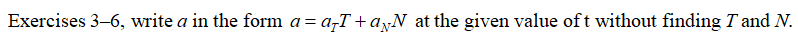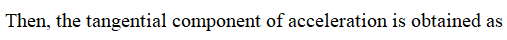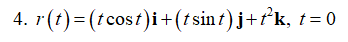# Exercises 3–6, write a in the form a = a TT + a NN at the given value of t without finding T and N.3. r(t) = (t + 1)i + 2tj + t2k, t = 14. r(t) = (t cos t)i + (t sin t)j + t2k,t=05. r(t) = t2i + (t + (1/3)t3 t = 0 )j + (t - (1/3)t3 ,t=06. r(t) = (et cos t)i + (et sin t)j + SQRT(2)et k, )k, t = 0

Question
2 views

Exercises 3–6, write a in the form a = a TT + a NN at the given value of t without finding T and N.

3. r(t) = (t + 1)i + 2tj + t2k, t = 1

4. r(t) = (t cos t)i + (t sin t)j + t2k,t=0

5. r(t) = t2i + (t + (1/3)t3 t = 0 )j + (t - (1/3)t3 ,t=0

6. r(t) = (et cos t)i + (et sin t)j + SQRT(2)et k, )k, t = 0

check_circle

Step 1Step 2Step 3...

### Want to see the full answer?

See Solution

#### Want to see this answer and more?

Solutions are written by subject experts who are available 24/7. Questions are typically answered within 1 hour.*

See Solution
*Response times may vary by subject and question.
Tagged in

### Derivative